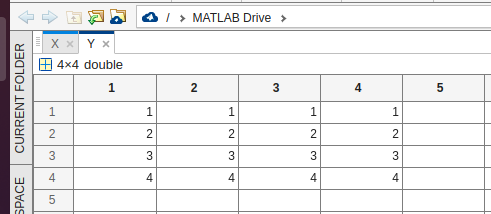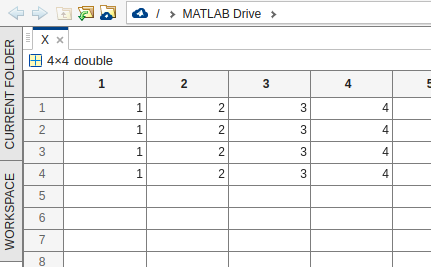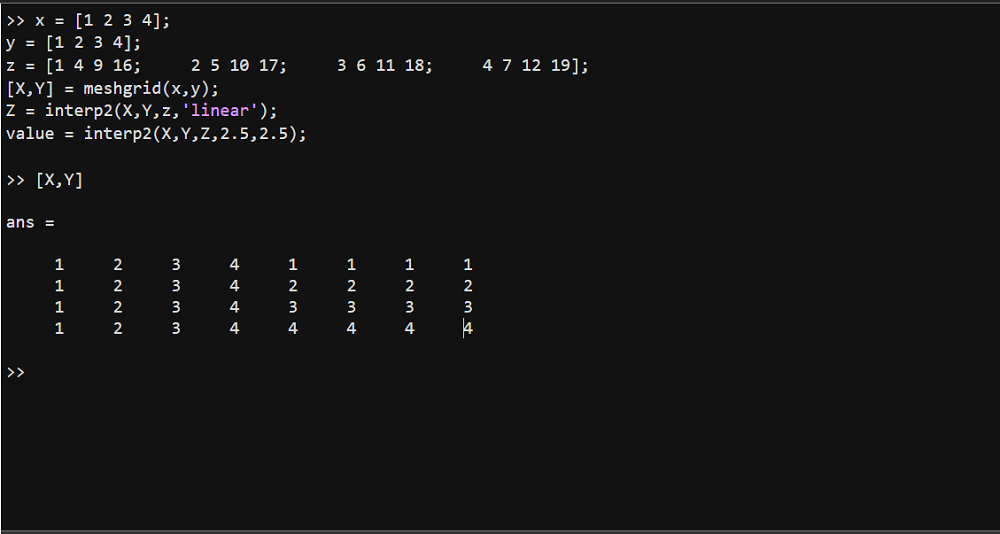GeeksforGeeks App
Open AppBrowser
Continue

# Double Interpolation using Lookup Tables in MATLAB

Double interpolation using lookup tables in MATLAB allows for efficient and accurate interpolation of data. This technique is useful when working with large datasets, as it can greatly reduce the amount of computation required to find interpolated values.

To perform double interpolation using lookup tables in MATLAB, we first need to create a lookup table from our dataset. This can be done using the interp2 function, which takes in the x and y coordinates of the data points, as well as the corresponding z values. For example, suppose we have the following dataset:

x = [1 2 3 4];
y = [1 2 3 4];
z = [1 4 9 16;  2 5 10 17;   3 6 11 18;    4 7 12 19];

We can create a lookup table from this data using the ‘interp2’ function as follows:

Example 1:

## Matlab

 `[X,Y] = meshgrid(x,y);``Z = interp2(X,Y,z,``'linear'``);`

The meshgrid function is used to create matrices of the x and y coordinates, which are then used as input to the interp2 function. The ‘linear’ option tells interp2 to use linear interpolation, which means that it will calculate the interpolated values by finding the straight line between the nearest data points.

Once we have our lookup table, we can use it to find interpolated values for any x and y coordinates within the range of our original data. To do this, we simply need to use the interp2 function again, this time providing the lookup table as input. For example, suppose we want to find the interpolated value at the coordinates (2.5,2.5). We can do this as follows:

## Matlab

 `value = interp2(X,Y,Z,2.5,2.5);`

This will return the interpolated value at the coordinates (2.5,2.5), which will be calculated using the lookup table we created earlier.

In summary, double interpolation using lookup tables in MATLAB allows for efficient and accurate interpolation of data. By creating a lookup table using the interp2 function, we can quickly and easily find interpolated values for any x and y coordinates within the range of our original data. This technique is particularly useful when working with large datasets, as it can greatly reduce the amount of computation required to find interpolated values.

Example 2:

## Matlab

 `% MATLAB Code``x = [1 2 3 4];``y = [1 2 3 4];``z = [1 4 9 16;     2 5 10 17;     3 6 11 18;     4 7 12 19];``[X,Y] = meshgrid(x,y);``Z = interp2(X,Y,z,``'linear'``);``value = interp2(X,Y,Z,2.5,2.5);`` ` `[X,Y];`

Output:My Personal Notes arrow_drop_up